Next: 7.3 Vectors in Discretized Up: 7. Numerical Considerations Previous: 7.1 Meshing

Subsections

# 7.2 Box Discretization

The discretization of the partial differential semiconductor equations in space and time is required to obtain difference equations which can be solved using numerical methods. A common approach for the discretization of the differential equations is the box discretization or box integration method [259,12], also known as the finite volume method.

## 7.2.1 Derivation

To apply the box discretization method, the mesh has to fulfill the Delaunay criterion and can therefore be split into boxes using a Voronoi tessellation . By applying the Voronoi tessellation a dual mesh is created so that every point in the domain is assigned to its closest vertex. All points which are closest to the same vertex form together an element of the dual mesh which is called box volume throughout this work (see Fig. 7.4). The transformation is bijective.

The box discretization method reformulates the equations based on this Voronoi tessellation and can be equally used in two- and three-dimensional simulation domains. Since the two-dimensional devices have a specified width which acts as a multiplier, all boxes are considered as volumes and the box boundary elements are considered as surfaces. The basic method of the box discretization concerns the divergence operator in the form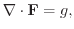(7.1)

whereand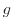are an arbitrary flux and generation term, respectively. The equation can be transformed by integration over a volume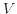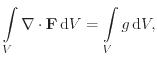(7.2)

and by applying Gauss's theorem,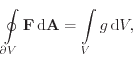(7.3)

where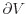is the surface of V and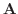is the outwardly oriented surface area vector. In the next step equation (7.3) is applied to each Voronoi box in the mesh (see Fig. 7.5). With this spatial quantization in boxes the two-dimensional boundaryis transferred to a polygon (in 2D) or polyhedron (in 3D) and can be split into planar surface elements perpendicular to the edges leading to the neighboring vertex. Every vertex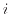has an associated box volume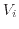and a set of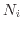neighboring boxes. Equation (7.3) can be approximated, i.e. discretized, for each vertex as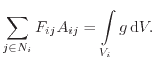(7.4)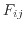is the flux through the boundary area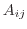between the boxesand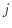(compare Fig. 7.5) and can be interpreted as the projection ofon the edge between the verticesandas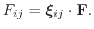(7.5)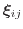is the unit vector pointing along edge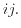From the discretization point of view,must be the value of the quantity at the mid-point of the edge which is exactly the boundary between the boxes. As will be shown in the next section, the proper discretization ofis relevant for numerically stable results and depends on the type of differential equation system used.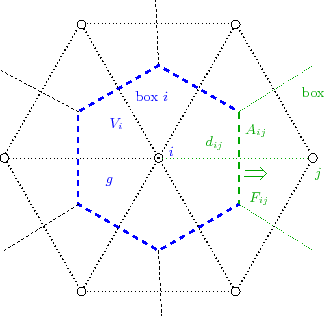The physical equations that describe semiconductor devices are laws of conservation. The derivation of the box discretization method is inherently conservative . The box discretization scheme is, therefore, widely used in semiconductor device simulations . In drift-diffusion simulations, the differential equations that have to be solved are Poisson's equation (4.1) and the current continuity equations (4.5) and (4.6). The flux quantitiesintroduced in (7.1) are the dielectric flux density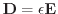for Poisson's equation and the current densities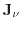for the continuity equation, respectively. The generation termsin (7.1) are the charge density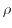and the carrier generation/recombination rates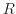/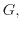respectively.

A considerable advantage of the box discretization method is that the only information required is the unstructured neighborhood information. This neighborhood information consists basically of two lists. First, a list of all boxes in the simulation domain, together with their center points (), i.e. the vertices, and the box volumes (). Second, a list of all edges connecting the vertices, together with the edge length (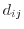), i.e. the distance between the vertices, and the common surface between the neighboring boxes (). It has to be noted that no more information about the elements is necessary for the evaluation of (7.4), which makes the box discretization independent of the box shape, including its dimensionality. This makes this scheme very straightforward to implement and a dimensional independent set-up of the simulator can be realized.

## 7.2.2 Discretization of Edges

The calculation of the one-dimensional projection of the flux on the edge depends on the type of the differential equation. For the dielectric flux density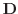in Poisson's equation the flux from boxto boxalong the connecting edge through the common boundary areais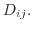This density is commonly approximated using the directional derivative of the electrostatic potential: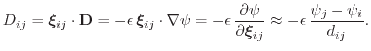(7.6)is the distance between the two vertices and the permittivity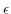is considered as a constant in each element. In MINIMOS-NT, for example, the permittivity is taken as the average between the permittivities associated with the verticesandasFor the current densitiesof the carrier typethe discretization of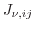is not as straightforward. Insertion of the drift-diffusion current relations from equations (4.7) and (4.8) in the continuity equations (4.5) and (4.6) results in a second order parabolic partial differential equation. Using a simple finite difference approach like in (7.6) leads to numerical oscillations if the drift term dominates over the diffusion term . Very fine meshes would be necessary to stabilize the system. A stable discretization can be obtained using the Scharfetter-Gummel method  instead. Here, the drift-diffusion current equations (4.7) and (4.8) are used to solve the one-dimensional carrier concentrations,and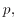respectively, along the edge. The boundary conditions of the carrier concentrations are given using the values at the corresponding verticesand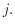The values of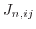and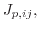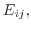and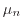and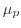are considered constant along the edge. Solving this one-dimensional differential equation results in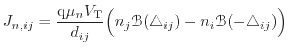(7.7)

for electrons and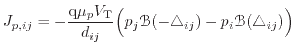(7.8)

for holes, where the temperature voltage is defined as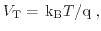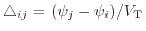andis the Bernoulli function defined as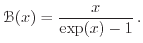(7.9)

## 7.2.3 Discretization of the Right Hand Side

Up to now, the generation termon the right hand side of (7.4), which is the charge densityin Poisson's equation and the carrier generation and recombination rate in the carrier equations, was only represented as an integral in continuum space. Most implementations calculate this integral by partitioning the box into pieces and adding the contributions to the integral of the box. Three different methods are considered in this work, each partitioning the Voronoi box differently. For the first assumption, the box is not split and the generation is considered constant over the whole box volume, as it is depicted in Fig. 7.6(a). This approach reduces the integral term of (7.4) to a simple product [12,262] which reads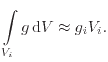(7.10)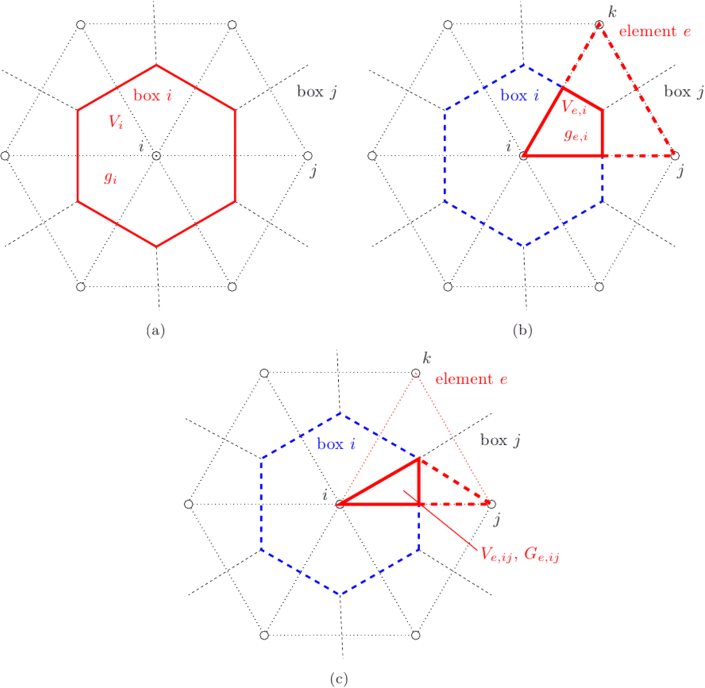This formulation perfectly fits to the box discretization method, because it only requires quantities which are stored on vertices and the only geometry information needed is the unstructured neighborhood information. This makes the assembling procedure of (7.10) very simple. MINIMOS-NT , for example, is one simulation tool using this technique. As will be discussed in Section 7.3, the calculation of vector quantities is somewhat more involved in this approach than for the other methods.

Another approach for assembling the generation integral is to assume the generation rate to be constant within one mesh element. In this case, the integral for one box volume is assembled using contributions from all adjacent elements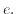Using the naming conventions from Fig. 7.6(b), this reads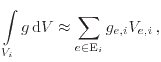(7.11)

where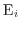is the set of all elements that contribute to the volume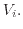In this scheme the generation term is estimated for each element (compare dashed red line in Fig. 7.6(b)), which might be a triangle, tetrahedra, box, or other mesh element. Its contributions can be used for all boxes the element is part of . This element-wise assembly results in an implicitly increased resolution and a more accurate physical representation . Some implementations associate different current densities for each edge pair (compare solid red line in Fig. 7.6(b)) and do not assume it to be constant within the whole element. In this case there are independent rates for each box the element is contained in. A method using this techniques is the edge-pair method .

The third approach in this classification is depicted in Fig. 7.6(c). This approach was presented for triangles only , but extensions to tetrahedra are possible. Here, each triangle is split into three different regions associated with the three edges. In each of them, a generation term is estimated, and two of them contribute to the sum of one box. Therefore, the summation for the generation integral of the box requires to consider two contributions per element:(7.12)

This approach was used by Laux et al.  in the context of calculating the impact-ionization rate. More on the calculations and the applied vector discretization in this approach will be given in Section 7.3.1. Note that this further splitting of the domain implicitly leads to a higher resolution and increased accuracy, which can be seen in the comparison in Section 7.3.3.

## 7.2.4 Limitations of the Box Discretization Method

The box method is used in most numerical device simulation environments as it is particle conservative and has proven to deliver good results, is numerically very stable, and is relatively simple to implement. Problems arise when the Delaunay criterion is violated. This leads to obtuse elements which degenerate the accuracy due to negative flux areas[267,264]. Also, use of the one-dimensional Scharfetter-Gummel discretization to solve multiple dimensional problems leads to the crosswind diffusion effect resulting in artificial current components perpendicular to the actual current direction . The accuracy of the discretization also degrades if triangles are aligned with the hypotenuse along the current flow. As depicted in Fig. 7.7, a vanishing boundary arealeads according to (7.4) to a vanishing contribution of the current along this edge. A zig-zag characteristic of the discretized current is the result. There have been many proposals for more accurate discretizations (e.g. Patil in ). Some focus on the extension of the one-dimensional to a two-dimensional Scharfetter-Gummel current discretization [269,270]. But none of these extensions is as universal to use as the box integration method which is dimension independent and can be used for structured and unstructured meshes alike.Next: 7.3 Vectors in Discretized Up: 7. Numerical Considerations Previous: 7.1 Meshing

O. Triebl: Reliability Issues in High-Voltage Semiconductor Devices